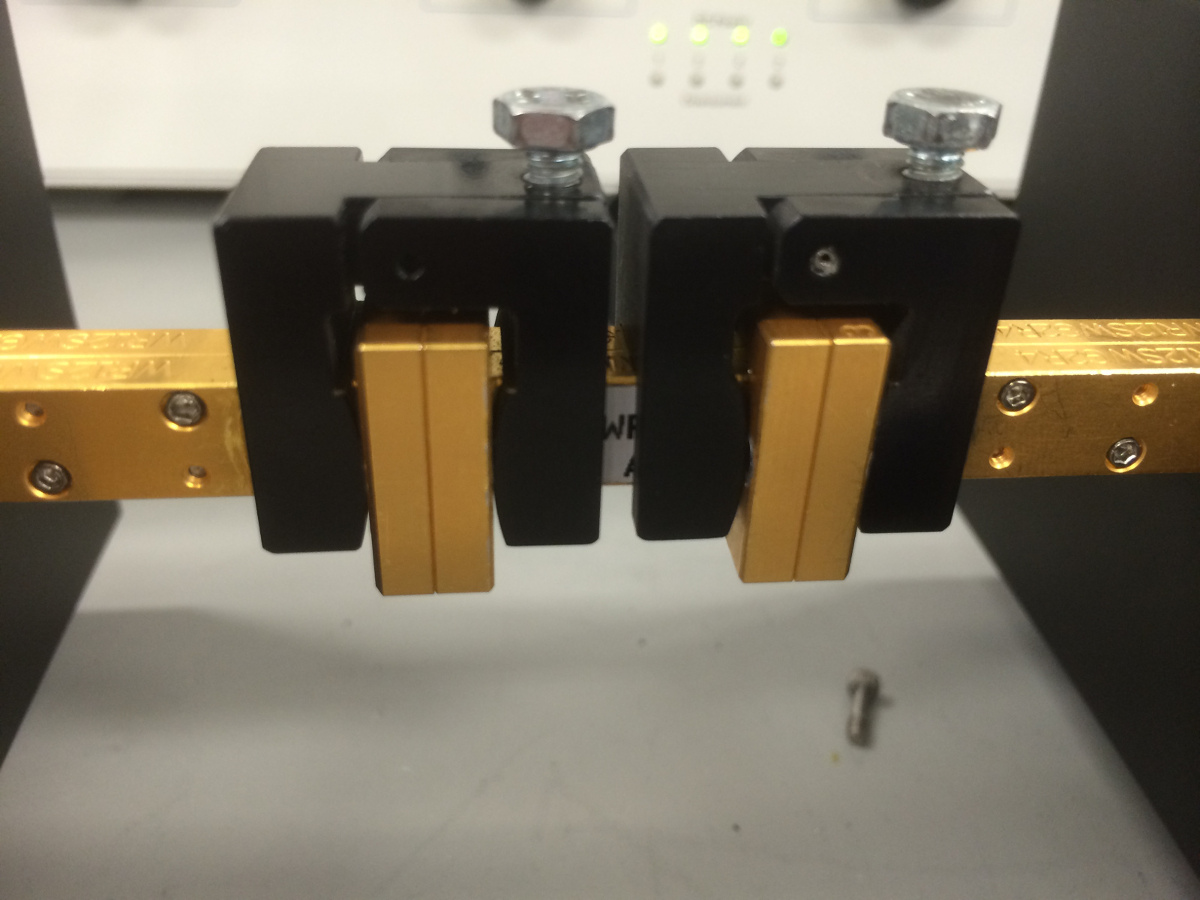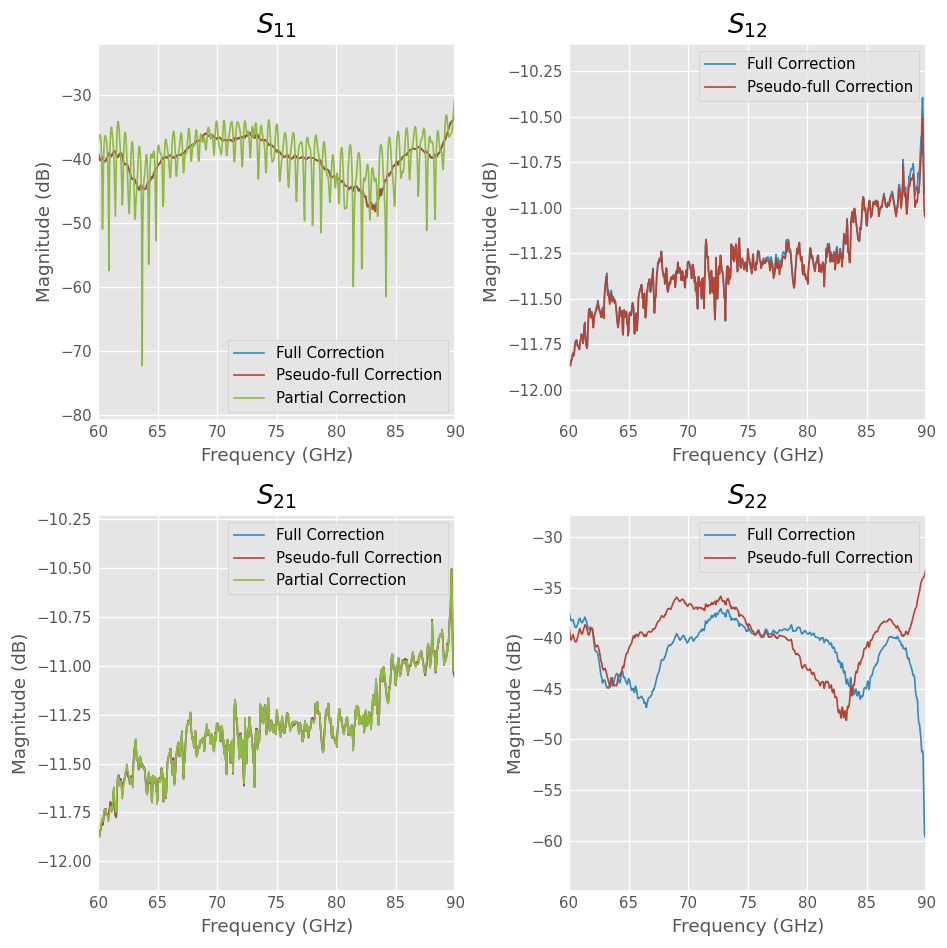# TwoPortOnePath, EnhancedResponse, and FakeFlip¶

## Intro¶

This example demonstrates a macgyver-ish shortcut you can take if you are measuring a device that is ** reciprocal** and symmetric on a switch-less three-receiver system. For more information about error correction this type of architecture, see Calibration With Three Receivers.

In general, full error correction of a 2-port network on a switchless three-receiver architecture requires each DUT to measured in two orientations. However, if the DUT is known to be reciprocal ($$S_{21}=S_{12}$$) and symmetric ($$S_{11}=S_{22}$$), then measurements in both orientations produce the same response, and therefore are unnecessary.

The following worked example compares the corrected response of a 10dB attenuator at WR-12 as corrected using full error correction and pseudo-full error correction using:

1. Full Correction

2. Pseudo-Full Correction (FakeFlip)

3. Partial (EnhancedResponse)

:

from IPython.display import *

:## Example¶

These measurements where taken on a Agilent PNAX with a set of VDI WR-12 TXRX-RX Frequency Extender heads. The measurements of the calibration standards and DUT’s were downloaded from the VNA by saving touchstone files of the raw s-parameter data to disk.

In the code that follows a TwoPortOnePath calibration is created from corresponding measured and ideal responses of the calibration standards. The measured networks are read from disk, while their corresponding ideal responses are generated using scikit-rf. More information about using scikit-rf to do offline calibrations can be found here.

:

import skrf as rf
%matplotlib inline
from pylab import *
rf.stylely()

from skrf.calibration import TwoPortOnePath
from skrf.media import RectangularWaveguide
from skrf import two_port_reflect as tpr
from skrf import  mil

# pull frequency information from measurements
frequency = raw['short'].frequency

# the media object
wg = RectangularWaveguide(frequency=frequency, a=120*mil, z0_override=50)

# list of 'ideal' responses of the calibration standards
ideals = [wg.short(nports=2),
tpr(wg.delay_short( 90,'deg'), wg.match()),
wg.match(nports=2),
wg.thru()]

# corresponding measurements to the 'ideals'
measured = [raw['short'],
raw['quarter wave delay short'],
raw['thru']]

# the Calibration object
cal = TwoPortOnePath(measured = measured, ideals = ideals )

/home/docs/checkouts/readthedocs.org/user_builds/scikit-rf/envs/latest/lib/python3.10/site-packages/skrf/calibration/calibration.py:1477: UserWarning: n_thrus is None, guessing which stds are transmissive
warn('n_thrus is None, guessing which stds are transmissive')


## Correction Options¶

With the calibration created above, we compare the corrected response of WR-12 10dB attenuator using Full, Pseudo-Full, and Partial Correction. Each correction algorithm is described below.

:

Image('three_receiver_cal/pics/symmetric DUT.jpg', width='75%')

:### Full Correction (TwoPortOnePath)¶

Full correction on this type of architecture has been called TwoPortOnePath. In scikit-rf using this correction algorithm requires the device to be measured in both orientations, forward and reverse, and passing them both to the apply_cal() function as a tuple. Neglecting the connector uncertainty, this type of correction is identical to full two-port SOLT calibration.

### Pseudo-full Correction ( FakeFlip)¶

If we assume the DUT is reciprocal and symmetric, then measuring the device in both orientations will produce the same result. Therefore, the reverse orientation measurement may be replaced by a copy of the forward orientation measurement. We refer to this technique as the Fake Flip.

Warning: Be sure that you understand the assumptions of reciprocity and symmetry before using this macgyver technique, incorrect usage can lead to nonsense results.

### Partial Correction (EnhancedResponse)¶

If you pass a single measurement to the apply_cal() function, then the calibration will employ partial correction. This type of correction is known as EnhancedResponse. While the Fake Flip technique assumes the device is reciprocal and symmetric, the EnhancedResponse algorithm implicitly assumes that the port 2 of the device is perfectly matched. The accuracy of the corrected result produced with either of these algorithms depends on accuracy of the assumptions.

## Comparison¶

:

dutf = raw['attenuator (forward)']
dutr = raw['attenuator (reverse)']

# note the correction algorithm is different depending on what is passed to
# apply_cal
corrected_full =     cal.apply_cal((dutf, dutr))
corrected_fakeflip = cal.apply_cal((dutf,dutf))
corrected_partial =  cal.apply_cal(dutf)

f, ax = subplots(2,2, figsize=(8,8))

for m in [0,1]:
for n in [0,1]:
ax_ = ax[m,n]
ax_.set_title('$S_{%i%i}$'%(m+1,n+1))
corrected_full.plot_s_db(m,n, label='Full Correction',ax=ax_ )
corrected_fakeflip.plot_s_db(m,n, label='Pseudo-full Correction', ax=ax_)
if n==0:
corrected_partial.plot_s_db(m,n, label='Partial Correction', ax=ax_)

tight_layout()

/home/docs/checkouts/readthedocs.org/user_builds/scikit-rf/envs/latest/lib/python3.10/site-packages/skrf/calibration/calibration.py:1943: UserWarning: only gave a single measurement orientation, error correction is partial without a tuple
warnings.warn('only gave a single measurement orientation, error correction is partial without a tuple')
/home/docs/checkouts/readthedocs.org/user_builds/scikit-rf/envs/latest/lib/python3.10/site-packages/skrf/mathFunctions.py:265: RuntimeWarning: divide by zero encountered in log10
out = 20 * npy.log10(z)[ ]: